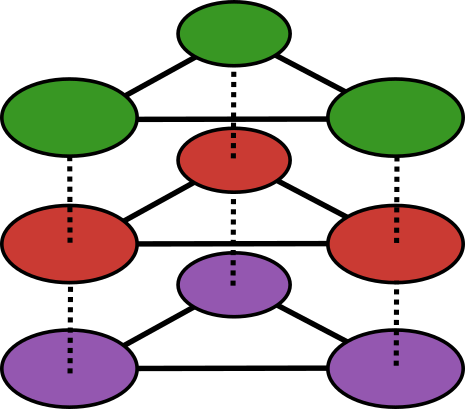## Julia Community 🟣# [ANN] MultilayerGraphs.jl: A Package to Construct, Handle and Analyse Multilayer Graphs

We are thrilled to announce MultilayerGraphs.jl: a Julia package for the construction, manipulation and analysis of multilayer graphs extending Graphs.jl.

MultilayerGraphs.jl provides two custom types, `MultilayerGraph` and `MultilayerDiGraph`, together with utilities to handle and analyse undirected and directed multilayer graphs implementing the mathematical formulation proposed by De Domenico et al. (2013).

The graphs composing the multilayer graph (i.e. layers and interlayers) can be of any type as long as they are proper extensions of `AbstractGraph{T}`. Then, since this package heavily relies on Graphs.jl and all of its extensions, it may also serve as a playground to test the overall status and consistency of the ecosystem API.

### Main Features

In the code block below we illustrate the main features of the package.

``````# Import necessary packages
using Graphs
using SimpleWeightedGraphs, MetaGraphs, SimpleValueGraphs
using MultilayerGraphs

# Set graph attributes
n_nodes   = 5        # Number of nodes
min_edges = n_nodes  # Minimum number of edges
max_edges = 10       # Maximum number of edges

# Define some graphs underlying layers and interlayers
simpledigraph         = SimpleDiGraph(n_nodes, rand(min_edges:max_edges))
simpleweighteddigraph = SimpleWeightedDiGraph(n_nodes, rand(min_edges:max_edges))
simplevalueoutgraph   = ValOutDiGraph( SimpleDiGraph(n_nodes,rand(min_edges:max_edges));
edgeval_types=(Int64, ),
edgeval_init=(s, d) -> (s + d, )
)

simplevaluedigraph    = ValDiGraph( SimpleDiGraph(n_nodes,rand(min_edges:max_edges));
edgeval_types=(Int64, ),
edgeval_init=(s, d) -> (s + d, )
)

# Collect all graphs in a vector
layer_graphs = [simpledigraph, simpleweighteddigraph, metadigraph, simplevalueoutgraph, simplevaluedigraph]

# Define layers
layers  = [ Layer(Symbol("layer_\$i"),     # Layer's name
graph;                    # Layer's underlying graph
U = Float64)              # Layer's adjacency matrix `eltype`
for (i,graph) in enumerate(layer_graphs)
]

# Define interlayers. Here we use the constructor for random interlayers.
## Note that the user does not need to specify all interlayers: the unspecified one s will be taken care of by the MultilayerDiGraph constructor, that will initialize them according to the a default interlayer type passed via the keyword argument `default_interlayer_type`
interlayers = [ Interlayer(n_nodes,                     # Number of nodes
:interlayer_layer_1_layer_2,  # Interlayer's name
:layer_1,                     # Source layer name
:layer_2,                     # Destination layer name
SimpleDiGraph{Int64},         # Underlying graph type
rand(min_edges:max_edges);    # Number of edges
U = Float64                   # Interlayers's adjacency matrix `eltype`
),
Interlayer(n_nodes, :interlayer_layer_1_layer_3,:layer_1, :layer_3, SimpleWeightedDiGraph{Int64}, rand(min_edges:max_edges); U = Float64 ), # Create another interlayer, the others will be automatically specified
]

# Define the MultilayerDiGraph
multilayerdigraph = MultilayerDiGraph(layers, interlayers)

# There are many other constructors for Layer, Interlayer and Multilayer(Di)Graph! Make sure to check them out in the documentation or in the REPL.

# Get all layers
multilayerdigraph.layers

# Get all Interlayers
multilayerdigraph.interlayers

add_edge!(multilayerdigraph, MultilayerVertex(1, :layer_1), MultilayerVertex(2, :layer_4)) # MultilayerVertex(1, :layer_1) refers to vertex 1 (i.e. the representation of node 1) in layer 1
## Check that the edge has been added
@assert has_edge(multilayerdigraph, MultilayerVertex(1, :layer_1), MultilayerVertex(2, :layer_4))
@assert multilayerdigraph.adjacency_tensor[1,2,1,4] == 1.0 # indexing is [vertex_1, vertex_2, vertex_1_layer_index, vertex_2_layer_index]

# Remove an edge
rem_edge!(multilayerdigraph, MultilayerVertex(1, :layer_1), MultilayerVertex(2, :layer_4))
## Check that the edge has been removed
@assert !has_edge(multilayerdigraph, MultilayerVertex(1, :layer_1), MultilayerVertex(2, :layer_4))
@assert multilayerdigraph.adjacency_tensor[1,2,1,4] == 0.0 # indexing is [vertex_1, vertex_2, vertex_1_layer_index, vertex_2_layer_index]

# Since Multilayer(Di)Graphs are extensions of Graphs.jl (https://juliagraphs.org/Graphs.jl/dev/ecosystem/interface/) all methods defined for AbstractGraph also work for Multilayer(Di)Graph.
## Below are some examples of multilayer-specific functions and of Graphs.jl's functions that had to be reimplemented anyway for technical reasons.

# Get the overlay monoplex graph
get_overlay_monoplex_graph(multilayerdigraph)

# Get the depth-weighted global clustering coefficient, with weights so that it coincides with the global clustering coefficient
wcc = multilayer_weighted_global_clustering_coefficient(multilayerdigraph, [1/3, 1/3, 1/3])
@assert wcc ≈ multilayer_global_clustering_coefficient(multilayerdigraph)

# Get the eigenvector centrality of each vertex and the relative error at each iteration of the algorithm that computes it
eig_centrality, errs = eigenvector_centrality( multilayerdigraph;
norm = "n",        # Normalization factor
tol = 1e-3         # Target relative inter-iteration error
)

# Get the modularity, given a clustering
modularity( multilayerdigraph,
rand([1, 2, 3, 4], length(nodes(multilayerdigraph)),length(multilayerdigraph.layers)) # Communities
)

# Von Neumann Entropy is currently implemented only for undirected multilayer graphs (i.e. for MultilayerGraph).
## You can find it in the tutorial included in the package documentation.
``````

### Future Developments

The package is currently under development and further steps would benefit enormously from the precious feedback of the JuliaGraph people, graph theorists, network scientists and all the users who might have general questions or suggestions.

Here we highlight the major future developments we have currently identified: